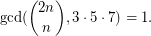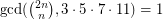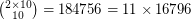# Divisibility of central binomial coefficients

 Importance: Medium ✭✭
 Author(s): Graham, Ronald L.
 Subject: Number Theory » Combinatorial Number Theory
 Keywords:
 Prize: \$1,000 each
 Posted by: maxal on: August 5th, 2007
Problem  (1)   Prove that there exist infinitely many positive integerssuch thatProblem  (2)   Prove that there exists only a finite number of positive integerssuch thatThe binomial coefficientis not divisible by primeiff all the base-digits ofare smaller thanIt has been conjectured that 1, 2, 10, 3159, and 3160 are the only positive numbers for whichholds.

* indicates original appearance(s) of problem.

### It seems Problem (1) was

It seems Problem (1) was solved last year, see http://arxiv.org/abs/1010.3070

### 2, 10, and 3159 are not valid for problem (2)

In the initial comment five values are stated to be not divisible by 3, 5, 7, and 11. That is true for 1 and 3160 but not for 2, 10, and 3159:

The last base-3-digit of 2 is 2. The last base-11-digit of 10 is 10. The last base-5-digit 3159 is 4.

Or check these facts:2 and 3159 are not in the sequence A030979 (mentioned in the bibliography).

### Reference for problem (2)

It appears that the right reference for question 2 should be A151750 instead.

### Problem 1 solved?

Looks like Problem 1 was solved by http://arxiv.org/PS_cache/arxiv/pdf/1010/1010.3070v1.pdf

### Does not look like it.

Does not look like it.